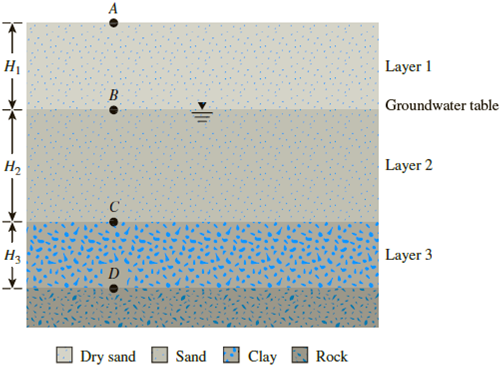Chapter 9, Problem 9.4PPrinciples of Geotechnical Enginee...

9th Edition
Braja M. Das + 1 other
ISBN: 9781305970939

Solutions

Chapter
SectionPrinciples of Geotechnical Enginee...

9th Edition
Braja M. Das + 1 other
ISBN: 9781305970939
Textbook Problem

Consider the soil profile in Problem 9.2. What is the change in effective stress at point C if:a. the water table drops by 2 m?b. the water table rises to the surface up to point A?c. the water level rises 3 m above point A due to flooding?Figure 9.25

(a)

To determine

Find the change in effective stress σ at point C when the water table drops by 2 m.

Explanation

Given information:

The thickness H1 of soil layer 1 is 5 m.

The thickness H2 of soil layer 2 is 8 m.

The thickness H3 of soil layer 3 is 3 m.

The void ratio (e) of soil in the first layer is 0.7.

The specific gravity Gs of the soil in the first layer is 2.69.

The void ratio (e) of soil in the second layer is 0.55.

The depth (h) of water table drop is 2 m.

Calculation:

Determine the dry unit weight γd of the soil in the first layer using the relation.

γd(layer1)=Gsγw1+e

Here, γw is the unit weight of the water.

Take the unit weight of the water as 9.81kN/m3.

Substitute 2.69 for Gs, 9.81kN/m3 for γw, and 0.7 for e.

γd(layer1)=2.69×9.811+0.7=15.52kN/m3

Determine the dry unit weight γd(layer2) of the soil in the second layer if the water table drops by 2 m using the relation.

γd(layer2)=Gsγw1+e

Substitute 2.7 for Gs, 9.81kN/m3 for γw, and 0.55 for e.

γd(layer2)=2.70×9.811+0.55=17.08kN/m3

Determine the saturated unit weight γsat of the soil in the second layer using the relation.

γsat(layer2)=γw(Gs+e)1+e

Substitute 9.81kN/m3 for γw, 2.7 for Gs, and 0.55 for e.

γsat(layer2)=9.81(2.7+0.55)1+0.55=20.57kN/m3

Calculate the total stress at point C (13 m) using the relation.

σ=γd×H1+γsat×H2

Substitute 15.52kN/m3 for γd, 5 m for H1, 20.57kN/m3 for γsat, and 8.0 m for H2.

σ=15.52×5.0+20.57×8.0=242.16kN/m2

Calculate the pore water pressure at point C (13 m) using the relation.

u=γw×H2

Substitute 9.81kN/m3 for γw and 8 m for H2

(b)

To determine

The change in effective stress σ at point C when the water rises to the surface up to point A.

(c)

To determine

Find the change in effective stress σ at point C when the water level rises 3 m above point A due to flooding.

Still sussing out bartleby?

Check out a sample textbook solution.

See a sample solution

The Solution to Your Study Problems

Bartleby provides explanations to thousands of textbook problems written by our experts, many with advanced degrees!

Get Started

What unit of measure is used to define cutting speeds?

Precision Machining Technology (MindTap Course List)

What is physical independence?

Database Systems: Design, Implementation, & Management

What types of injuries can occur to the ears during welding?

Welding: Principles and Applications (MindTap Course List)